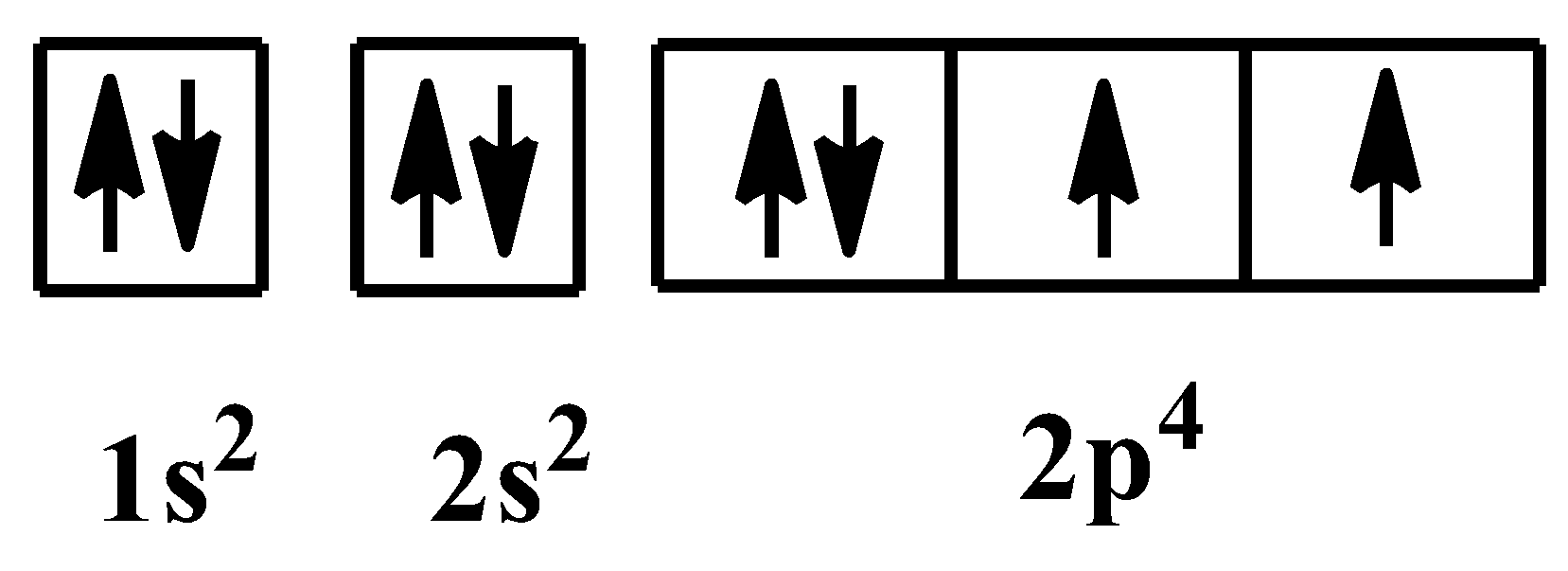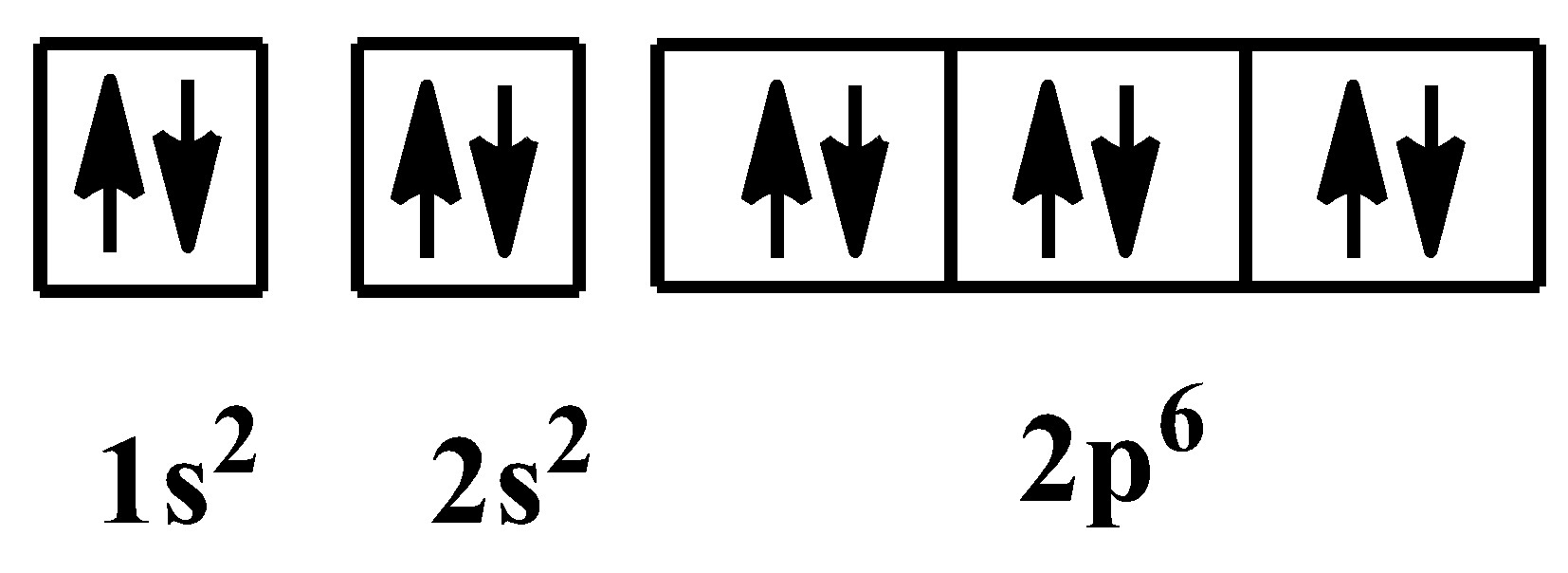QuestionAnswers

# What is the electronic configuration of ${{O}^{2-}}$ ion?Verified
129k+ views
Hint: Write the electronic configuration of oxygen atom in its neutral state. Then add two electrons to the outermost orbital of the configuration.

For writing the electronic configuration of any element, we have to know its atomic number. The atomic number of an element is the number of protons present inside the nucleus of its atom or the number of electrons present inside a neutral atom of that element.
On the periodic table, the element oxygen occupies the position at the crossing of the second period and sixteenth group. The atomic number of oxygen is “$8$”. So, its electronic configuration is as below:
$1{{s}^{2}}2{{s}^{2}}2{{p}^{4}}$
The orbital arrangement of electrons for the above configuration is shown below:As you can see, there are two unpaired electrons in the valence subshell of the oxygen element. In${{O}^{2-}}$ion, there are two extra electrons which means, the unpaired electrons are paired now. So the electronic configuration becomes:
$1{{s}^{2}}2{{s}^{2}}2{{p}^{6}}$
This is the electronic configuration of neon, which is a noble gas. The orbital arrangement for the above configuration is as shown below: